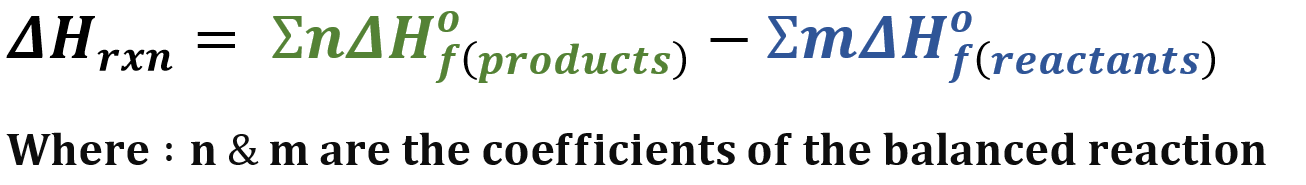# Problem: Calculate ΔH° for each of the following reactions, which occur in the atmosphere.a. C2H4 (g) + O3 (g) → CH3CHO (g) + O2 (g)

###### FREE Expert Solution

The enthalpy of reaction can be calculated using the equation below:Now we need the enthalpy of formation for each compound present. We need to look for that in standard tables (books).

92% (89 ratings)###### Problem Details

Calculate ΔH° for each of the following reactions, which occur in the atmosphere.

a. C2H4 (g) + O3 (g) → CH3CHO (g) + O2 (g)